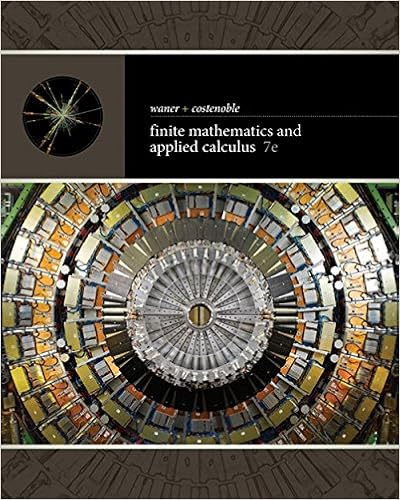Final Exam - Final Exam Laurence Field Math 152 Section 31...

• CountAtomAardvark3912
• 11
• 100% (1) 1 out of 1 people found this document helpful

This preview shows page 1 - 4 out of 11 pages.

We have textbook solutions for you!
The document you are viewing contains questions related to this textbook.The document you are viewing contains questions related to this textbook.
Chapter 6 / Exercise 23
Finite Mathematics and Applied Calculus
Costenoble/WanerExpert Verified
Final Exam Laurence Field Math 152, Section 31 March 12, 2012 Name: Instructions: This exam has 8 questions for a total of 120 points. The value of each part of each question is stated. The time allowed is two hours. Partial credit may be given for incorrect answers if your working is legible. No books, notes or calculators are allowed. Since there are no calculators, give your answers in exact form. No decimal approximation is needed. Important: For question 8, answer EITHER part 8(a) OR part 8(b). [Your score for question 8 will be the greater of your 8(a) score and your 8(b) score.] Answer all parts of questions 1–7. Question Points Score 1 15 2 15 3 15 4 15 5 15 6 15 7 15 8 15 Total: 120 i
We have textbook solutions for you!
The document you are viewing contains questions related to this textbook.The document you are viewing contains questions related to this textbook.
Chapter 6 / Exercise 23
Finite Mathematics and Applied Calculus
Costenoble/WanerExpert Verified
1. Compute the following integrals. (a) 5 Z x x x + 1 dx (b) 5 Z tan(3 x ) dx (c) 5 Z π/ 2 - π/ 2 sin 5 x + cos x dx 1
2. Consider the function f ( x ) = e 1 /x . (a) 1 Is f even, odd or neither? (b) 8 Find all local extrema, points of inflection, and horizontal and vertical asymptotes of the graph of f . (c) 4 Sketch the graph of f , showing all the features you found in (b). (d) 2 Write a formula for f - 1 ( y ).
•••